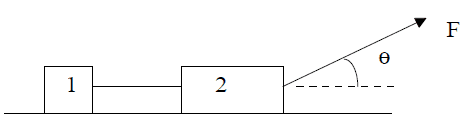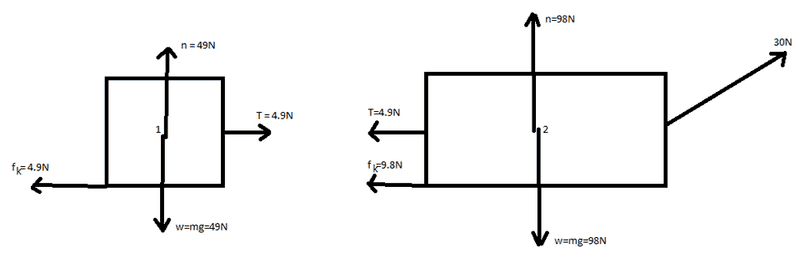# Force on two boxes attached by a string

## Homework Statement

Two blocks connected by a light string are being pulled to the right by a constant force of
magnitude 30.0 N making an angle of 25o from the horizontal. The coefficient of kinetic friction
between each block and the surface is 0.100. If m1 = 5.00 kg and m2 = 10.0 kg , (a) Draw a free body diagram for each object. (b) Find the accleration of the system. (c) Find the tension in the string.F=ma

## The Attempt at a Solution

My attempt at part a:My attempt at part b:

I got that the force in the x direction of block 2 being pulled is 27.2. So I got that the sum of the forces would be: 27.2-9.8-4.9 = 12.5

Since F=ma and m equals 15, I got that the acceleration equals .833m/s², but that is not the correct answer.

Edit: OK I think I have figured out part b. I realized that the 30N force on m2 was causing a force of 12.7N in the y direction, making the normal force on m2 equal to 85.3N, making the kinetic friction on m2 equal to 8.53N. So why you sum up the forces in the x direction you get: 27.2-8.53-4.9 = 13.77. I then divided by the total weight which is 15 and got .918. Is this correct?

My attempt at part c:
I am really not sure how begin to solve part c

Last edited:

Doc Al
Mentor
Edit: OK I think I have figured out part b. I realized that the 30N force on m2 was causing a force of 12.7N in the y direction, making the normal force on m2 equal to 85.3N, making the kinetic friction on m2 equal to 8.53N. So why you sum up the forces in the x direction you get: 27.2-8.53-4.9 = 13.77. I then divided by the total weight which is 15 and got 9.18. Is this correct?
Yes, all good.

Hint: The tension is an unknown force. In your FBDs, you show the tension force as 4.9 N! Just show it as unknown T. (And, you realize, that your value for the normal force for m2 is incorrect. In the diagram, I'd just show the normal forces as N1 and N2. You'll figure them out as needed.)

My attempt at part c:
I am really not sure how begin to solve part c
Revise your FBDs as suggested above. Use the acceleration to figure out the tension. Hint: Apply Newton's 2nd law to an individual block.

Ok I think I figured out part c. I used F=ma so F=(5)(.918)=4.59. I then added the friction on block 1 and got 9.49N. Is this correct?

And for the free-body diagrams, I don't need any of the actual values? I was never really sure about this so I just filled them in.

Doc Al
Mentor
Ok I think I figured out part c. I used F=ma so F=(5)(.918)=4.59. I then added the friction on block 1 and got 9.49N. Is this correct?
Yes, that's correct. I would describe it like this:
ΣF = ma
T - 4.9 = (5)(.918) = 4.59
T = 9.49 N

And for the free-body diagrams, I don't need any of the actual values? I was never really sure about this so I just filled them in.
The purpose of the free body diagram is to help you analyze forces and solve for the unknowns. (That's why it was the first part of this problem.) If you already knew the values, you'd wouldn't need a diagram--the problem would already be solved.

Oh ok yeah that makes sense.

Thanks for all of your help! I think I am finally starting to understand some of this stuff.